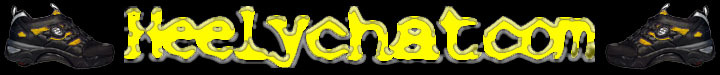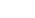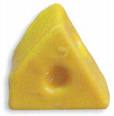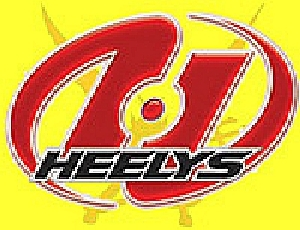FAQ  Search  Memberlist  Heelypedia
Author Message
 parker=coolPosted: Thu Mar 15, 2007 6:54 pmJoined: 13 Feb 2007 Posts: 221 Location: Austin, TX
 does anyone know of a good skatepark in austin, TX?? _________________I could say somthing funny here, but I'm not going to.WIYHPosted: Thu Aug 23, 2007 9:42 pmJoined: 07 Jun 2007 Posts: 150 Location: Southwest
 i saw one when i was in austin a few weeks ago but i couldn't tell u what street or anything _________________I GOTS 5,050 GHETTO POINTS! =~=~=~=~=~=~=~=~=~=~=~=~=~=~=~=~=~ [Sun 2:25] Homicidal Poptart: just gettting in touch with the more feminem side of me (that didnt sound gay did it) =~=~=~=~=~=~=~=~=~=~=~=~=~=~=~=~=~ [Sun 14:30] heelzthatkillz: i cant stop it feels so good!!!!! =~=~=~=~=~=~=~=~=~=~=~=~=~=~=~=~=~ CrAzYhEeLyEr711 (11:06:25 PM): but dude his boxers were hot =~=~=~=~=~=~=~=~=~=~=~=~=~=~=~=~=~WIYHPosted: Thu Aug 23, 2007 9:56 pmJoined: 07 Jun 2007 Posts: 150 Location: Southwest
 _________________I GOTS 5,050 GHETTO POINTS! =~=~=~=~=~=~=~=~=~=~=~=~=~=~=~=~=~ [Sun 2:25] Homicidal Poptart: just gettting in touch with the more feminem side of me (that didnt sound gay did it) =~=~=~=~=~=~=~=~=~=~=~=~=~=~=~=~=~ [Sun 14:30] heelzthatkillz: i cant stop it feels so good!!!!! =~=~=~=~=~=~=~=~=~=~=~=~=~=~=~=~=~ CrAzYhEeLyEr711 (11:06:25 PM): but dude his boxers were hot =~=~=~=~=~=~=~=~=~=~=~=~=~=~=~=~=~parker=coolPosted: Mon Nov 05, 2007 10:09 amJoined: 13 Feb 2007 Posts: 221 Location: Austin, TX
 cool, thanks. Do you live in Texas? _________________I could say somthing funny here, but I'm not going to.Display posts from previous: All Posts1 Day7 Days2 Weeks1 Month3 Months6 Months1 Year Oldest FirstNewest First
 All times are GMT - 5 Hours

 Page 1 of 1

 Jump to: Select a forum General Discussion----------------The ExaltedGeneralPicture & VideoOff-TopicTips Tricks & TechniquesCommerceGamesCesspit Where to Heel----------------Northeast USSoutheast USMidwest/Central USNorthwest USSouthwest USUnited KingdomCanadaEverywhere Else
You cannot post new topics in this forum
You cannot reply to topics in this forum
You cannot edit your posts in this forum
You cannot delete your posts in this forum
You cannot vote in polls in this forum